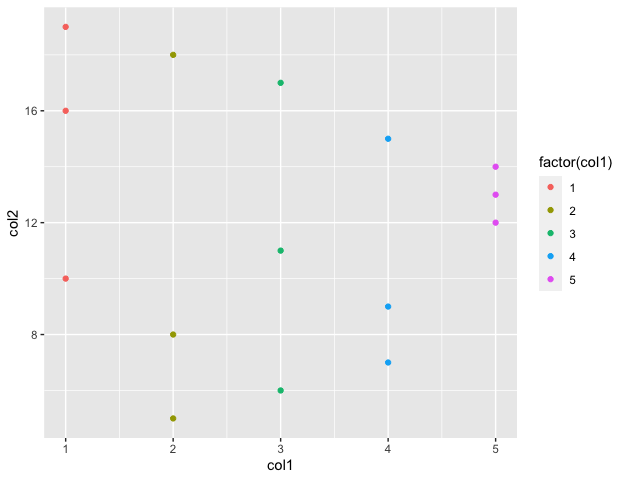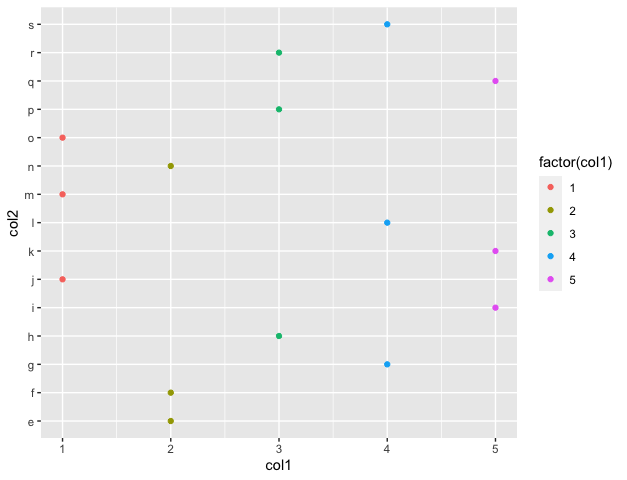# Create a Scatter Plot with Multiple Groups using ggplot2 in R

• Last Updated : 24 Oct, 2021

In this article, we will discuss how to create a scatter plot with multiple groups in R Programming Language.

Geoms can be added to the plot to compute various graphical representations of the data in the plot (points, lines, bars). The geom_point() method is used to create scatter plots in R. The geoms may also be specified with the additional mappings, like color to differently color the points pertaining to different groups.

`geom_point(aes(color = ))`

## R

 `library``(``"ggplot2"``)`` ` ` ` `# creating a data frame``df < - ``data.frame``(col1=``sample``(``rep``(``c``(1: 5), each=3)),``                  ``col2=5: 19)``print``(``"original dataframe"``)``print``(df)`` ` `# plotting the data``ggplot``(df, ``aes``(x=col1, y=col2)) +``geom_point``(``aes``(color=``factor``(col1)))`

Output

``` "original dataframe"
col1 col2
1     2    5
2     3    6
3     4    7
4     2    8
5     4    9
6     1   10
7     3   11
8     5   12
9     5   13
10    5   14
11    4   15
12    1   16
13    3   17
14    2   18
15    1   19```Explanation: The groups are created according to the differences in values of col1. All the circles, for example, belonging to col1=1 are given the red color. This is also illustrated in the index of the plot.

The following code snippet indicates the method where one of the data frame columns is a non-integral one :

## Python3

 `library(``"ggplot2"``)`` ` `# creating a data frame``df < ``-` `data.frame(col1``=``sample(rep(c(``1``: ``5``), each``=``3``)),``                  ``col2``=``letters[``5``:``19``])`` ` `print``(``"original dataframe"``)``print``(df)`` ` `# plotting the data``ggplot(df, aes(x``=``col1, y``=``col2)) ``+``geom_point(aes(color``=``factor(col1)))`

Output

``` "original dataframe"
col1 col2
1     2    e
2     2    f
3     4    g
4     3    h
5     5    i
6     1    j
7     5    k
8     4    l
9     1    m
10    2    n
11    1    o
12    3    p
13    5    q
14    3    r
15    4    s```My Personal Notes arrow_drop_up